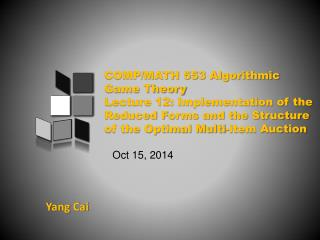Download PresentationYang Cai

Yang Cai

Télécharger la présentationYang Cai

- - - - - - - - - - - - - - - - - - - - - - - - - - - E N D - - - - - - - - - - - - - - - - - - - - - - - - - - -
Presentation Transcript

1. COMP/MATH 553 Algorithmic Game TheoryLecture 12: Implementation of the Reduced Forms and the Structure of the Optimal Multi-item Auction Oct 15,2014 YangCai

2. New Decision Variables Variables: Interim Allocation rule aka. “REDUCED FORM”: * : Pr( ) πij(vi) valuation vi valuation vi j i i i * :E[ pricei ]

3. A succinct LP • Variables: • πij(vi): probability that item jis allocated to bidder iif her reported valuation (bid) is viin expectation over every other bidders’ valuations (bids); • pi(vi) :price bidder ipays if her reported valuation (bid) is viin expectation over every other bidder’s valuations (bids) • Constraints: • BIC: for all vi and v’i in Ti • IR: for all vi in Ti • Feasibility: exists an auction with this reduced form. • Objective: • Expected revenue:

4. Implementation of a Feasible Reduced Form • After solving the succinct LP, we find the optimal reduced form π* and p*. • Can you turn π* and p* into an auction whose reduced form is exactly π* and p*? • This is crucial, otherwise being able to solve the LP is meaningless. • Will show you a way to implement any feasible reduced form, and it reveals important structure of the revenue-optimal auction!

5. Implementation of a Feasible Reduced Form

6. Set of Feasible Reduced Forms • Reduced form is collection ; • Can view it as a vector ; • Let’s call set of feasible reduced forms ; • Claim 1:F(D) is a convex polytope. • Proof: Easy! • A feasible reduced form is implemented by a feasible allocation rule M. • M is a distribution over deterministic feasible allocation rules, of which there is a finite number. So: , where is deterministic. • Easy to see: • So, F(D) = convex hull of reduced forms of feasible deterministic mechanisms

7. Set of FeasibleReduced Forms F(D) ? Q: Is there a simpleallocation rule implementing the corners?

8. * Is there a simple allocation rule implementing a corner? F(D) virtual welfare maximizing interim rule when virtual value functions are the fi’s ------ (1) expected virtual welfare of an allocation rule with interim rule π’ --- (2) interpretation: virtual value derived by bidder iwhen given item j when his type is A

9. Is there a simple allocation rule implementing a corner? F(D) virtual welfare maximizing interim rule when virtual value functions are the fi’s ? • Q: Can you name an algorithm doing this? A: YES, the VCG allocation rule ( w/ virtual value functions fi, i=1,..,m ) = : virtual-VCG( { fi } ) interpretation: virtual value derived by bidder iwhen given item j when his type is A

10. Characterization Theorem [C.-Daskalakis-Weinberg] F(D) How about implementing any point insideF(D)? F(D) is a Convex Polytopewhose corners are implementable by virtual VCG allocation rules.

11. Carathéodory’s theorem If some point x is in the convex hull of P then For example: x = ¼(0,1) + ¼(1,0) +½(0,0) Carathéodory’sTheorem: If a point x of Rd lies in the convex hull of a set P, there is a subset P′ of P consisting of d + 1 or fewer points such that x lies in the convex hull of P′.

12. Characterization Theorem [C.-Daskalakis-Weinberg] F(D) Any point inside F(D) is a convex combination (distribution) over the corners. The interim allocation rule of any feasible mechanism can be implemented as a distribution over virtual VCG allocation rules.

13. Structure of the Optimal Auction

14. Characterization of Optimal Multi-Item Auctions Theorem [C.-Daskalaks-Weinberg]: Optimal multi-item auction has the following structure: • Bidders submit valuations (t1,…,tm) to auctioneer. • Auctioneer samples virtual transformations f1,…, fm • Auctioneer computes virtual types t’i = fi(ti) • Virtual welfare maximizing allocation is chosen. Namely, each item is given to bidder with highest virtual value for that item (if positive) • Prices are charged to ensure truthfulness.

15. Characterization of Optimal Multi-Item Auctions Theorem [C.-Daskalaks-Weinberg]: Optimal multi-item auction has the following structure: • Bidders submit valuations (t1,…,tm) to auctioneer. • Auctioneer samples virtual transformations f1,…, fm • Auctioneer computes virtual types t’i = fi(ti) • Virtual welfare maximizing allocation is chosen. Namely, each item is given to bidder with highest virtual value for that item (if positive) • Prices are charged to ensure truthfulness. • Exact same structure as Myerson! • in Myerson’s theorem: virtual function = deterministic • here, randomized (and they must be)

16. Interesting Open Problems • Another difference: in Myerson’s theorem: virtual function is given explicitly, in our result, the transformation is computed by an LP. Is there any structure of our transformation? • In single-dimensional settings, the optimal auction is DSIC. In multi-dimensional settings, this is unlikely to be true. What is the gap between the optimal BIC solution and the optimal DSIC solution?